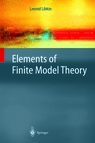##Elements of Finite Model Theory

### Leonid Libkin

From the back cover:
The book is an introduction to finite model theory that stresses computer science origins of the area. In addition to presenting the main techniques for analyzing logics over finite models, the book deals extensively with applications in databases, complexity theory, and formal languages, as well as other branches of computer science. It covers Ehrenfeucht-Fraïssé games, locality-based techniques, complexity analysis of logics, including the basics of descriptive complexity, second-order logic and its fragments, connections with finite automata, fixed point logics, finite variable  logics, zero-one laws, embedded finite models, and gives a brief tour of recently discovered applications of finite model theory.
This book can be used both as an introduction to the subject, suitable for a one- or two-semester graduate course, or as reference for researchers who apply techniques from logic in computer science.

 Table of contents Introduction Preliminaries Ehrenfeucht-Fraisse Games Locality and Winning Games Ordered Structures Complexity of First-Order Logic Monadic Second-Order Logic and Automata Logics with Counting Turing Machines and Finite Models Fixed Point Logics and Complexity Classes Finite variable logics Zero-one laws Embedded Finite Models Other applications of finite model theory.           Bibliography, List of Notation, Index, Name Index. Ordering:

Download table of contents and a sample chapter                            Errata